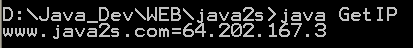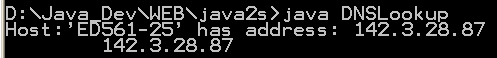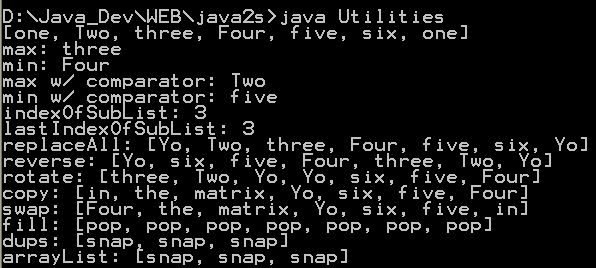# A class that performs some subnet calculations given a network address and a subnet mask. : IP Address « Network Protocol « Java

A class that performs some subnet calculations given a network address and a subnet mask.

```
/*
* Licensed to the Apache Software Foundation (ASF) under one or more
* contributor license agreements.  See the NOTICE file distributed with
* this work for additional information regarding copyright ownership.
* The ASF licenses this file to You under the Apache License, Version 2.0
* (the "License"); you may not use this file except in compliance with
* the License.  You may obtain a copy of the License at
*
*      http://www.apache.org/licenses/LICENSE-2.0
*
* Unless required by applicable law or agreed to in writing, software
* distributed under the License is distributed on an "AS IS" BASIS,
* WITHOUT WARRANTIES OR CONDITIONS OF ANY KIND, either express or implied.
* See the License for the specific language governing permissions and
* limitations under the License.
*/

import java.util.regex.Matcher;
import java.util.regex.Pattern;

/**
* A class that performs some subnet calculations given a network address and a subnet mask.
* @see http://www.faqs.org/rfcs/rfc1519.html
* @author <rwinston@apache.org>
* @since 2.0
*/
public class SubnetUtils {

private static final String IP_ADDRESS = "(\\d{1,3})\\.(\\d{1,3})\\.(\\d{1,3})\\.(\\d{1,3})";
private static final String SLASH_FORMAT = IP_ADDRESS + "/(\\d{1,3})";
private static final Pattern addressPattern = Pattern.compile(IP_ADDRESS);
private static final Pattern cidrPattern = Pattern.compile(SLASH_FORMAT);
private static final int NBITS = 32;

private int netmask = 0;
private int address = 0;
private int network = 0;
private int broadcast = 0;

/**
* Constructor that takes a CIDR-notation string, e.g. "192.168.0.1/16"
* @param cidrNotation A CIDR-notation string, e.g. "192.168.0.1/16"
*/
public SubnetUtils(String cidrNotation) {
calculate(cidrNotation);
}

/**
* Constructor that takes two dotted decimal addresses.
* @param address An IP address, e.g. "192.168.0.1"
* @param mask A dotted decimal netmask e.g. "255.255.0.0"
*/
public SubnetUtils(String address, String mask) {
calculate(toCidrNotation(address, mask));
}

/**
* Convenience container for subnet summary information.
*
*/
public final class SubnetInfo {
private SubnetInfo() {}

private int netmask()       { return netmask; }
private int network()       { return network; }
private int address()       { return address; }
private int broadcast()     { return broadcast; }
private int low()           { return network() + 1; }
private int high()          { return broadcast() - 1; }

public boolean isInRange(String address)    { return isInRange(toInteger(address)); }
private boolean isInRange(int address)      { return ((address-low()) <= (high()-low())); }

public String getBroadcastAddress()         { return format(toArray(broadcast())); }
public String getNetworkAddress()           { return format(toArray(network())); }
public String getNetmask()                  { return format(toArray(netmask())); }
public String getAddress()                  { return format(toArray(address())); }
public String getLowAddress()               { return format(toArray(low())); }
public String getHighAddress()              { return format(toArray(high())); }
public int getAddressCount()                { return (broadcast() - low()); }

public int asInteger(String address)        { return toInteger(address); }

public String getCidrSignature() {
return toCidrNotation(
format(toArray(address())),
format(toArray(netmask()))
);
}

public String[] getAllAddresses() {
String[] addresses = new String[getAddressCount()];
for (int add = low(), j=0; add <= high(); ++add, ++j) {
addresses[j] = format(toArray(add));
}
return addresses;
}
}

/**
* Return a {@link SubnetInfo} instance that contains subnet-specific statistics
* @return
*/
public final SubnetInfo getInfo() { return new SubnetInfo(); }

/*
* Initialize the internal fields from the supplied CIDR mask
*/
private void calculate(String mask) {
Matcher matcher = cidrPattern.matcher(mask);

if (matcher.matches()) {
address = matchAddress(matcher);

/* Create a binary netmask from the number of bits specification /x */
int cidrPart = rangeCheck(Integer.parseInt(matcher.group(5)), 0, NBITS-1);
for (int j = 0; j < cidrPart; ++j) {
netmask |= (1 << 31-j);
}

/* Calculate base network address */
network = (address & netmask);

/* Calculate broadcast address */
broadcast = network | ~(netmask);
}
else
throw new IllegalArgumentException("Could not parse [" + mask + "]");
}

/*
* Convert a dotted decimal format address to a packed integer format
*/
private int toInteger(String address) {
Matcher matcher = addressPattern.matcher(address);
if (matcher.matches()) {
return matchAddress(matcher);
}
else
throw new IllegalArgumentException("Could not parse [" + address + "]");
}

/*
* Convenience method to extract the components of a dotted decimal address and
* pack into an integer using a regex match
*/
private int matchAddress(Matcher matcher) {
int addr = 0;
for (int i = 1; i <= 4; ++i) {
int n = (rangeCheck(Integer.parseInt(matcher.group(i)), 0, 255));
addr |= ((n & 0xff) << 8*(4-i));
}
return addr;
}

/*
* Convert a packed integer address into a 4-element array
*/
private int[] toArray(int val) {
int ret[] = new int;
for (int j = 3; j >= 0; --j)
ret[j] |= ((val >>> 8*(3-j)) & (0xff));
return ret;
}

/*
* Convert a 4-element array into dotted decimal format
*/
private String format(int[] octets) {
StringBuilder str = new StringBuilder();
for (int i =0; i < octets.length; ++i){
str.append(octets[i]);
if (i != octets.length - 1) {
str.append(".");
}
}
return str.toString();
}

/*
* Convenience function to check integer boundaries
*/
private int rangeCheck(int value, int begin, int end) {
if (value >= begin && value <= end)
return value;

throw new IllegalArgumentException("Value out of range: [" + value + "]");
}

/*
* Count the number of 1-bits in a 32-bit integer using a divide-and-conquer strategy
* see Hacker's Delight section 5.1
*/
int pop(int x) {
x = x - ((x >>> 1) & 0x55555555);
x = (x & 0x33333333) + ((x >>> 2) & 0x33333333);
x = (x + (x >>> 4)) & 0x0F0F0F0F;
x = x + (x >>> 8);
x = x + (x >>> 16);
return x & 0x0000003F;
}

/* Convert two dotted decimal addresses to a single xxx.xxx.xxx.xxx/yy format
* by counting the 1-bit population in the mask address. (It may be better to count
* NBITS-#trailing zeroes for this case)
*/
private String toCidrNotation(String addr, String mask) {
return addr + "/" + pop(toInteger(mask));
}
}

```

### Related examples in the same category

 1 Get IP 2 Get all IP addresses 3 Convert a hostname to the equivalent IP address4 Getting the Hostname of an IP Address 5 Get canonical host name 6 Getting the IP Address and Hostname of the Local Machine 7 Perform Network Lookup with the InetAddress class 8 Get hostname by a byte array containing the IP address 9 Display multiple IP addresses 10 Looking Up the Address of a Host11 Retrieve the hostname of an IP Address 12 Retrieve the IP address of a hostname 13 Report By Address 14 Determine the IP address and hostname of the local machine 15 An nslookup clone in Java 16 Looking for Ports: 0 -- 1024 17 Looking for Port: 1024 -- 65536 18 Looking for Port: start from 65535 19 Resolves string ip address and returns host name as a string 20 Resolves hostname and returns ip address as a string 21 Resolves ip address and returns host name as a string 22 This program demonstrates the InetAddress class 23 Check if IP address match pattern 24 Converts IPv4 address in its textual presentation form into its numeric binary form. 25 Convert IPv6 presentation level address to network order binary form. 26 Finds this computer's global IP address 27 Finds a local, non-loopback, IPv4 address 28 Check if the specified address is a valid numeric TCP/IP address 29 Check if the specified address is within the required subnet 30 Check if the specified address is a valid numeric TCP/IP address and return as an integer value 31 Convert a raw IP address array as a String 32 Determine if the given string is a valid IPv4 or IPv6 address.33 A string input validator for IPs and domains. 34 Get Current Environment Network IP